Next: Boundary Conditions for and Up: Magnetostatics in Magnetic Media Previous: Magnetic Susceptibility and Permeability

# Ferromagnetism

There is, however, a third class of magnetic materials called ferromagnetic materials. Such materials are characterized by a possible permanent magnetization, and generally have a profound effect on magnetic fields (i.e.,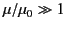). Unfortunately, ferromagnetic materials do not exhibit a linear dependence between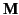and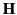, or between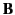and, so that we cannot employ Equations (691) and (692) with constant values of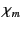and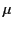. It is still expedient to use Equation (692) as the definition of, with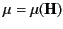. However, this practice leads to complications under certain circumstances. In fact, the permeability of a ferromagnetic material, as defined by Equation (692), can vary through the entire range of possible values from zero to infinity, and may be either positive or negative. The most sensible approach is to consider each problem involving ferromagnetic materials separately, try to determine which region of the-diagram is important for the particular case in hand, and then make approximations appropriate to this region.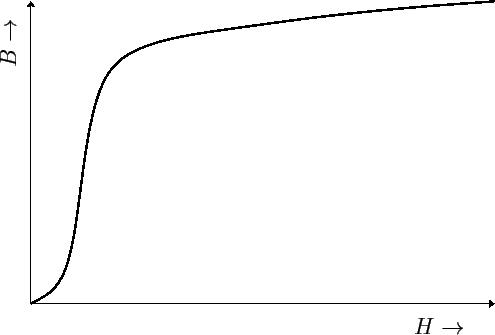Let us, first, consider an unmagnetized sample of ferromagnetic material. If the magnetic intensity, which is initially zero, is increased monotonically, then the-relationship traces out a curve such as that shown in Figure 2. This is called a magnetization curve. It is evident that the permeabilitiesderived from the curve (according to the rule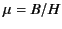) are always positive, and show a wide range of values. The maximum permeability occurs at the knee'' of the curve. In some materials this maximum permeability is as large as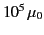. The reason for the knee in the curve is that the magnetizationreaches a maximum value in the material, so that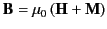(693)

continues to increase at largeonly because of the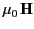term. The maximum value ofis called the saturation magnetization of the material.

Next, consider a ferromagnetic sample magnetized by the previously described procedure. If the magnetic intensityis decreased then the-relation does not follow back down the curve of Figure 2, but instead moves along a new curve, shown in Figure 3, to the point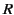. The magnetization, once established, does not disappear with the removal of. In fact, it takes a reversed magnetic intensity to reduce the magnetization to zero. Ifcontinues to build up in the reversed direction then(and, hence,) becomes increasingly negative. Finally, ifincreases again then the operating point follows the lower curve of Figure 3. Thus, the-curve for increasingis quite different to that for decreasing. This phenomenon is known as hysteresis.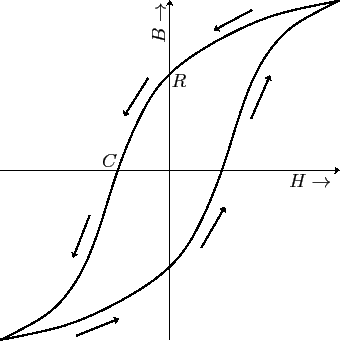The loop shown in Figure 3 is called the hysteresis loop of the material in question. The value ofat the pointis called the retentivity or remanence. The magnitude ofat the point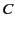is called the coercivity. It is evident thatis negative in the second and fourth quadrants of the loop, and positive in the first and third quadrants. The shape of the hysteresis loop depends not only on the nature of the ferromagnetic material, but also on the maximum value ofto which the material has been subjected. However, once this maximum value,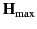, becomes sufficient to produce saturation in the material, the hysteresis loop does not change shape with any further increase in.

Ferromagnetic materials are used either to channel magnetic flux (e.g., around transformer circuits), or as sources of magnetic field (e.g., permanent magnets). For use as a permanent magnet, the material is first magnetized by placing it in a strong magnetic field. However, once the magnet is removed from the external field, it is subject to a demagnetizing. Thus, it is vitally important that a permanent magnet should possess both a large remanence and a large coercivity. As will become clear, later on, it is generally a good idea for the ferromagnetic materials used to channel magnetic flux around transformer circuits to possess small remanences and small coercivities.Next: Boundary Conditions for and Up: Magnetostatics in Magnetic Media Previous: Magnetic Susceptibility and Permeability
Richard Fitzpatrick 2014-06-27